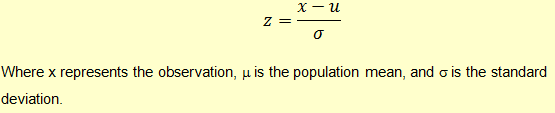# Standard Normal Distribution

A normal distribution can be described using just two parameters, namely (μ), mean and variance (σ2).

In a normal distribution, these two variables could take any value. For example, for a normally distributed stock portfolio, the mean could be 10% and the standard deviation could be 20%.

A standard normal distribution is a standardized form of normal distribution with a mean μ = 0 and standard deviation σ = 1.

We can standardize any normal random variable, by computing a z-score for it. z-scores make it easier to compare data values measured on different scales. A z-score reflects how many standard deviations above or below the mean a raw score is. The z-score is positive if the data value lies above the mean and negative if the data value lies below the mean. Z-score is represented using the following formula:Where x represents the observation, m is the population mean, and s is the standard deviation.

# Checkout our eBooks and Templates

eBooks and templates related to finance, R programming, Python, and Excel.
Visit Store
Get our Data Science for Finance Bundle for just $29$51. That's 43% OFF.
Get it for $51$29